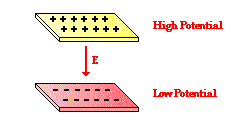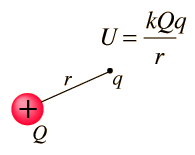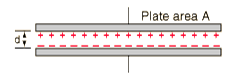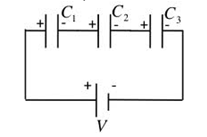Click to Chat

1800-1023-196

+91-120-4616500

CART 0

• 0

MY CART (5)

Use Coupon: CART20 and get 20% off on all online Study Material

ITEM
DETAILS
MRP
DISCOUNT
FINAL PRICE
Total Price: Rs.

There are no items in this cart.
Continue Shopping• Complete JEE Main/Advanced Course and Test Series
• OFFERED PRICE: Rs. 15,900
• View Details

Revision Notes on Electrostatic Potential and Capacitance

Electric Potential:-

(a) Electric potential, at any point, is defined as the negative line integral of electric field from infinity to that point along any path.

(b) V(r) = kq/r

(c)  Potential difference, between any two points, in an electric field is defined as the work done in taking a unit positive charge from one point to the other against the electric field.

WAB = q [VA-VB]

So, V = [VA-VB] = W/q

Units:- volt (S.I), stat-volt (C.G.S)Dimension:- [V] = [ML2T-3A-1]

Relation between volt and stat-volt:- 1 volt = (1/300) stat-volt

Relation between electric field (E) and electric potential (V):-

E = -dV/dx = --dV/dr

Potential due to a point charge:-

V = (1/4π ε0) (q/r)

Potential at point due to several charges:-

V = (1/4π ε0) [q1/r1 + q2/r2 + q3/r3]

= V1+V2+ V2+….

Potential due to charged spherical shell:-

(a) Outside, Vout = (1/4π ε0) (q/r)

(b) Inside, Vin = - (1/4π ε0) (q/R)

(c) On the surface, Vsurface = (1/4π ε0) (q/R)

Potential due to a uniformly charged non-conducting sphere:-

(a) Outside, Vout = (1/4π ε0) (q/r)

(b) Inside, Vin = (1/4π ε0) [q(3R2-r2)/2R3]

(c) On the surface, Vsurface = (1/4π ε0) (q/R)

(d) In center, Vcenter = (3/2) [(1/4π ε0) (q/R)] = 3/2 [Vsurface]

Common potential (two spheres joined by thin wire):-

(a) Common potential, V = (1/4π ε0) [(Q1+Q2)/(r1+r2)]

(b) q1 = r1(Q1+Q2)/(r1+r2) = r1Q/ r1+r2 ;  q2 =  r2Q/ r1+r2

(c) q1/q2 = r1/r2 or σ1/ σ2 = r1/r2

Potential at any point due to an electric dipole:-

V (r,θ) = qa cosθ/4πε0r2 = p cosθ/4πε0r2

(a) Point lying on the axial line:- V = p/4πε0r2

(b) Point situated on equatorial lines:- V = 0

If n drops coalesce to form one drop, then,

(a) R = n1/3r

(b) Q = nq

(c) V = n2/3Vsmall

(d) σ = n1/3 σsmall

(e) E = n1/3 Esmall

Electric potential energy U or work done of the system W having charge q1 and q2:-W = U = (1/4πε0) (q1q2/r12) = q1V1

Electric potential energy U or work done of the system W of a three particle system having charge q1, q2 and q3:-

W = U = (1/4πε0) (q1q2/r12 + q1q3/r13 + q2q3/r23)

Electric potential energy of an electric dipole in an electric field:- Potential energy of an electric dipole, in an electrostatic field, is defined as the work done in rotating the dipole from zero energy position to the desired position in the electric field.

?

(a) If θ = 90º, then W = 0

(b) If θ = 0º, then W = -pE

(c) If θ = 180º, then W = pE

Kinetic energy of a charged particle moving through a potential difference:-

K. E = ½ mv2 = eV

Conductors:- Conductors are those substance through which electric charge easily.

Insulators:- Insulators (also called dielectrics) are those substances through which electric charge cannot pass easily.

Capacity:- The capacity of a conductor is defined as the ratio between the charge of the conductor to its potential

C = Q/V

Units:-

Dimension of C:- [M-1L-2T4A2]

Capacity of an isolated spherical conductor:-

C = 4πε0r

Capacitor:- A capacitor or a condenser is an arrangement which provides a larger capacity in a smaller space.

Capacity of a parallel plate capacitor:-Cair = ε0A/d

Cmed = Kε0A/d

Here, A is the common area of the two plates and d is the distance between the plates.

Effect of dielectric on the capacitance of a capacitor:-

C = ε0A/[d-t+(t/K)]

Here d is the separation between the plates, t is the thickness of the dielectric slab A is the area and K is the dielectric constant of the material of the slab.

If the space is completely filled with dielectric medium (t=d), then,

C = ε0KA/ d

Capacitance of a sphere:-

(a) Cair = 4πε0R

(b) Cmed = K (4πε0R)

Capacity of a spherical condenser:-

(a) When outer sphere is earthed:-

Cair = 4πε0 [ab/(b-a)]

Cmed = 4πε0 [Kab/(b-a)]

(b) When the inner sphere is earthed:-

C1= 4πε0 [ab/(b-a)]

C2 = 4πε0b?

Net Capacity, C '=4πε0[b2/b-a]

Increase in capacity, ΔC = 4π ε0

It signifies, by connecting the inner sphere to earth and charging the outer one we get an additional capacity equal to the capacity of outer sphere.

Capacity of a cylindrical condenser:-

Cair = λl / [(λ/2π ε0) (loge b/a)] = [2π ε0l /(loge b/a) ]

Cmed = [2πKε0l /(loge b/a) ]

Potential energy of a charged capacitor (Energy stored in a capacitor):-

W = ½ QV = ½ Q2/C = ½ CV2

Energy density of a capacitor:-

U = ½ ε0E2 = ½ (σ2/ ε0)

This signifies the energy density of a capacitor is independent of the area of plates of distance between them so long the value of E does not change.

Grouping of Capacitors:-

(a)

(i) Capacitors in parallel:- C = C1+C2+C3+…..+Cn ?The resultant capacity of a number of capacitors, connected in parallel, is equal to the sum of their individual capacities.

(ii)V1= V2= V3 = V

(iii) q1 =C1V, q2 = C2V,  q3 = C3V

(iv) Energy Stored, U = U1+U2+U3

(b)

(i) Capacitors in Series:- 1/C = 1/C1 + 1/ C2 +……+ 1/Cn?The reciprocal of the resultant capacity of a number of capacitors, connected in series, is equal to the sum of the reciprocals of their individual capacities.

(ii) q1 = q2 = q3 = q

(iii) V1= q/C1, V2= q/C2, V3= q/C3

(iv) Energy Stored, U = U1+U2+U3

Energy stored in a group of capacitors:-

(a) Energy stored in a series combination of capacitors:-

W = ½ (q2/C1) + ½ (q2/C2) + ½ (q2/C3) = W1+W2+W3

Thus, net energy stored in the combination is equal to the sum of the energies stored in the component capacitors.

(b) Energy stored in a parallel combination of capacitors:-

W = ½ C1V 2 +½ C2V 2 + ½ C3V 2 = W1+W2+W3

The net energy stored in the combination is equal to sum of energies stored in the component capacitors.

Force of attraction between plates of a charged capacitor:-

(a) F = ½ ε0E2A

(b) F = σ2A/2ε0

(c) F=Q2/2ε0A

Force on a dielectric in a capacitor:-

F = (Q2/2C2) (dC/dx) = ½ V2 (dC/dx)

Common potential when two capacitors are connected:-

V = [C1V1+ C2V2] / [C1+C2] = [Q1+Q2]/ [C1+C2]

Charge transfer when two capacitors are connected:-

ΔQ = [C1C2/C1+C2] [V1-V2]

Energy loss when two capacitors are connected:-

ΔU = ½ [C1C2/C1+C2] [V1-V2] 2

Charging of a capacitor:-

(a) Q = Q0(1-e-t/RC)

(b) V = V0(1-e-t/RC)

(c) I = I0(1-e-t/RC)

(d) I0 = V0/R

Discharging of a capacitor:-

(a) Q = Q0(e-t/RC)

(b) V = V0(e-t/RC)

(c)  I = I0(e-t/RC)

Time constant:-### Course Features

• 728 Video Lectures
• Revision Notes
• Previous Year Papers
• Mind Map
• Study Planner
• NCERT Solutions
• Discussion Forum
• Test paper with Video Solution